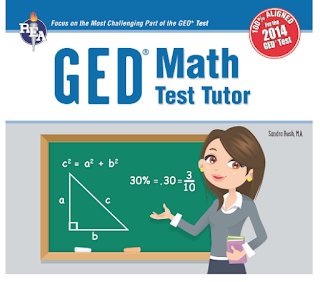## Labels

### Next-Generation GED Math Test OverviewMs. Sandra Rush M.A., author

Skills necessary to be successful with the GED Mathematical Reasoning test:
1. math skills
2. calculator skills
3. some computer skills
The latest version of the GED math test is called "Mathematical Reasoning". Previously, the GED test emphasized mathematical skills, knowing mathematical facts and formulas, and how to perform specific calculations for a problem. Starting in 2014, the emphasis is on understanding and logical thinking rather than memorization, and it is based on real-world problem-solving skills. According from Sandra Rush in her book, the next-generation test expects the test-taker to understand what a problem is asking for and proceed to answer it based on the information given. Use the four-part plan for reasoning skills to answer the questions:
1. Figure out what is known, what is necessary to solve the problem, what information is missing, and what is unnecessary.
2. Devise a strategy to solve the problem. This may involve making a sketch or a table.
3. Solve the problem according to your strategy.
4. Make sure the answer makes sense.
The GED Math Reasoning test measures how well you can figure out what is asked for and then apply mathematical skills, whether from your own memory bank or by using the tools that are available on the test, to come up with answers.

## Test time:The total test time is 90 minutes, but that includes 2 minutes for an introduction and wrap-up, so the actual testing time is only 88 minutes.

## Test point:

The test is worth 50 points, but there aren't necessarily 50 questions. Some questions are worth twice as much as others - that is because they are twice as difficult. Also, one chart, table, or problem scenario may have several questions associated with it. The computer uses a split screen for this type of question. On a split screen, the graphic or scenario stays on the left side of the screen, and the questions appear one by one on  the right screen. Fortunately, there is a "Previous" button if any of these questions depend at all on a response to prior question.

## Components:Fifty-five percent of the questions involve algebra, but that doesn't mean you are just given an equation and asked to solve it. Questions on any of the math subjects on the test may involve algebraic reasoning.

## Type of questions:

1. Multiple choice
2. Drop-down
3. Fill-in-the-blank
4. Hot-Spot items
5. Drag-and-drop